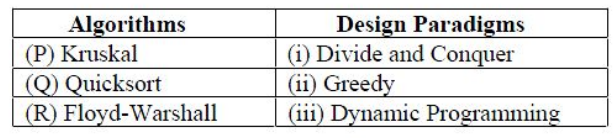# GATE | GATE-CS-2017 (Set 1) | Question 29

Consider the following tableMatch the algorithm to design paradigms they are based on:
(A) P-(ii), Q-(iii), R-(i)
(B) P-(iii), Q-(i), R-(ii)
(C) P-(ii), Q-(i), R-(iii)
(D) P-(i), Q-(ii), R-(iii)

Explanation:

• Kruskal’s  is a greedy technique of Minimum Spanning Tree Algorithm to find an edge of the least possible weight that connects any two trees in the forest.

• QuickSort is a Divide and Conquer algorithm. It picks an element as pivot and partitions the given array around the picked pivot.
• Floyd Warshall Algorithm is for solving the All Pairs Shortest Path problem using Dynamic Programming. The problem is to find shortest distances between every pair of vertices in a given edge weighted directed Graph.

My Personal Notes arrow_drop_up
Article Tags :

Be the First to upvote.

Please write to us at contribute@geeksforgeeks.org to report any issue with the above content.